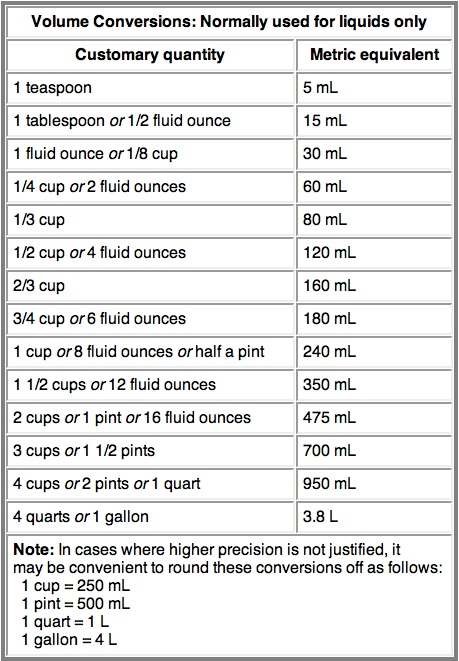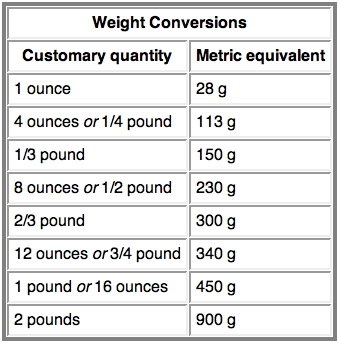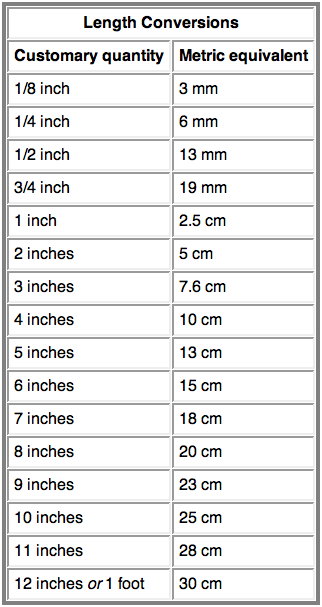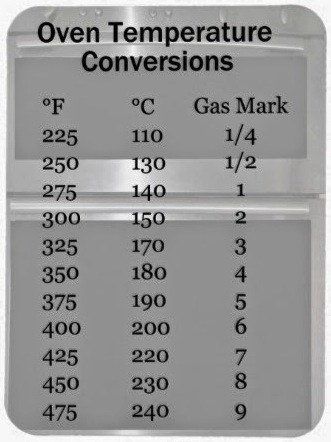# metric conversion chart

Nov 12, 2014

## Metric Conversion Chart

PLEASE NOTE: I NEVER EXPECTED SO MANY INTERNATIONAL VISITORS AND HAD NO EXPERIENCE WITH MEASURING BY WEIGHT. THE GENERALLY ACCEPTED STANDARD FOR FLOUR IS LISTED IN BLUE AND HOW IT WEIGHS IN MY KITCHEN IN RED.

Flour
1 cup  =  4 1/4 ounces =  Generally Accepted 120 grams – When I weigh I get 125-130 grams

1/2 cup  =  2  1/8  ounces =  60 grams / 63-65 grams

1/3 cup  =  1 1/2 ounces  =  40 grams / 42-43 grams

1/4 cup  =  1.05  ounces  =  30 grams / 31-32.5 grams

Granulated & Caster Sugar
1 cup  =  7 ounces  =  200 grams

1/2 cup  =  3.5 ounces  =  100 grams

1/3 cup  =  2.3 ounces  =  66 grams

1/4 cup  =  1.75 ounces  =  50 grams

1 Tablespoon =  0.45 ounces  =  12.50 grams

Yeast
1 packet = 7 grams

1 teaspoon = 3 1/2 grams

1/4 teaspoon = 1 gram

Powdered Sugar & Powdered Cocoa
1 cup  =  4 ounces  =  114 grams

1/2 cup  =  1.75 ounces  =  50 grams

1/3 cup  =  1.15 ounces  =  33 grams

1/4 cup  =  .85 ounces  =  25 grams

1 Tablespoon =  0.20 ounces  =  6.25 grams

Liquids
1 cup  =  8 ounces  =  240 mL

1/2 cup  =  4 ounces  =  120 mL

1/3 cup  =  2.6 ounces  =  80 mL

1/4 cup  =  2 ounces  =  60 mL

1 Tablespoon =  0.50 ounce  =  15 mL

Butter
1 Tablespoon  =  0.50 ounce  =  14 grams

1/4 cup  =  2 ounces  =  56 grams

1/3 cup  =  2.6 ounces  =  75 grams

1/2 cup  =  4 ounces  =  113 grams

1 cup  =  8 ounces  =  226 grams

Oven Baking Temperatures
225 degrees F  =  110 degrees C  =  1/4 gas number
250 degrees F  =  130 degrees C  =  1/2 gas number
275 degrees F  =  140 degrees C  =  1 gas number
300 degrees F  =  150 degrees C  =  2 gas number
325 degrees F  =  165 degrees C  =  3 gas number
350 degrees F  =  180 degrees C  =  4 gas number
375 degrees F  =  190 degrees C  =  5 gas number
400 degrees F  =  200 degrees C  =  6 gas number
425 degrees F  =  220 degrees C  =  7 gas number
450 degrees F  =  230 degrees C  =  8 gas number
475 degrees F  =  245 degrees C  =  9 gas number
500 degrees F  =  260 degrees C  =  10 gas number

…Below are all the charts I had perviously posted, compiled from other sources…

Liquids (and Herbs & Spices)WeightLength (1 cm = 10 mm)Temperature